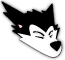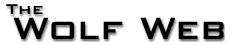User not logged in - login - register
 Home Calendar Books School Tool Photo Gallery Message Boards Users Statistics Advertise Site Info
go to bottom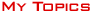|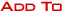|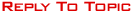Message Boards » » Calculus Question Page 
 HockeyRomanAll American11811 Postsuser infoedit post Integrating Separable Differential Equations:For this, K is a constant. And the original DiffEq is dV/dt=K*V^1/2I integrate it just fine and get 2V^-1/2=K(t+C)Here's my question: When I distribute the K, it gets "absorbed" by the C, right? At which point you plug in your initial conditions to find C for your solution. But that sets up V=(Kt+C/2)^2. So why doesn't the C take in the 1/2 as well?More aptly, I have an issue (a separate problem)with 2*(h)^1/2=(-1/163)t+C, the solution showed the 2 not becoming C/2, but rather k, thus giving the solution h(0)=(k)^2. I'm stumped.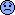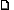10/26/2012 10:37:44 PM simonnbest gottfriend28968 Postsuser infoedit post regarding the first problem: you can absorb the 1/2 into C if you haven't solved for C yet. if you use an IC to find a value for C immediately after you integrate, then you have to carry the 1/2 (b/c the value you should have found is 1/2 the value that you did find).in the second problem: if you solve that for h(t) you geth(t) = (1/(4*163**2))(t**2) - (C/163)(t) + C**2 (where the 1/2 is absorbed into the C),so it looks like your IC is supposed to find that C=k, in which case h(0) = k**2.10/26/2012 11:21:12 PM HockeyRomanAll American11811 Postsuser infoedit post The problem I see is that depending on when you distribute the 1/2 or plug in your initial conditions, it changes the answer for C, and I am not sure which one is correct. :-/10/27/2012 12:12:17 PM lewisjeAll American9195 Postsuser infoedit post First, you had a typo: The correct answer is 2V^(1/2)=K*(t+C), and when you divide both sides by 2 you get V^(1/2)=(K/2)*(t+C).(You had another typo when you typed "Kt+C/2" because that literally means "the sum of Kt and half C" when you meant (Kt+C)/2, or "half the sum of Kt and C.")Anyway, you shouldn't "absorb" the parameter K into the constant of integration, so when you distribute, you get V^(1/2)=Kt/2+KC/2, and the answer in terms of V is V=(Kt/2+KC/2)^2, but it's more easily written as V=(K^2/4)(t+C)^2Here's why you shouldn't absorb that parameter in, using the example in which V(1)=1: The general solution as written gives 1=(K^2/4)(1+C)^2, so that C=2/K-1; however, if you had decided to distribute and "absorb," the general "solution" would look like V=(Kt/2+C)^2, from which 1=(K/2+C)^2, so that C=1-K/2.Now this looks okay, because the "new C" is just KC/2, but if K=0, the proper form of the general solution gives V=0, while the improper form gives V=C^2, which doesn't actually solve the differential equation unless C=0.tl;dr: It's okay to "absorb" specific numbers or constants known to be nonzero that are factors to constants of integration, but not parameters that could be zero, like K.(In particular, the C *does* take in the 1/2.)10/27/2012 1:10:13 PM HockeyRomanAll American11811 Postsuser infoedit post But K isn't zero. It's actually -.00028164661745409.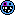It was just easier to separate and integrate by naming it "K".10/27/2012 1:45:22 PM The E ManSuspended15268 Postsuser infoedit post lol weather math10/27/2012 6:45:41 PM HockeyRomanAll American11811 Postsuser infoedit post Don't hate! Haha10/27/2012 9:04:44 PM lewisjeAll American9195 Postsuser infoedit post oh oh ohso it's not some parameter for a family of differential equationsk, well the reason that your answer for C is different based on whether you absorb or don't is that factor, like if C is after absorbing, then C=2C/KUsually when I write this stuff out, I use different letters for different variants of the "arbitrary constant"; as an example, when finding the general solution to dy/dx=y, I start with ln|y|=x+C but end up with y=Ae^x, where A={0 if y=0, e^C if y>0, -e^C if y<0}, and it's much easier to reason about than just calling it "y=Ce^x" by using C for both purposes.10/28/2012 1:40:21 AM catalystAll American8704 Postsuser infoedit post maths itt10/29/2012 10:29:30 AM HockeyRomanAll American11811 Postsuser infoedit post It got a 100 on the project!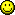11/30/2012 3:31:45 PM
Message Boards » Study Hall » Calculus Question Page 
go to top||Admin Options : move topic | lock topic